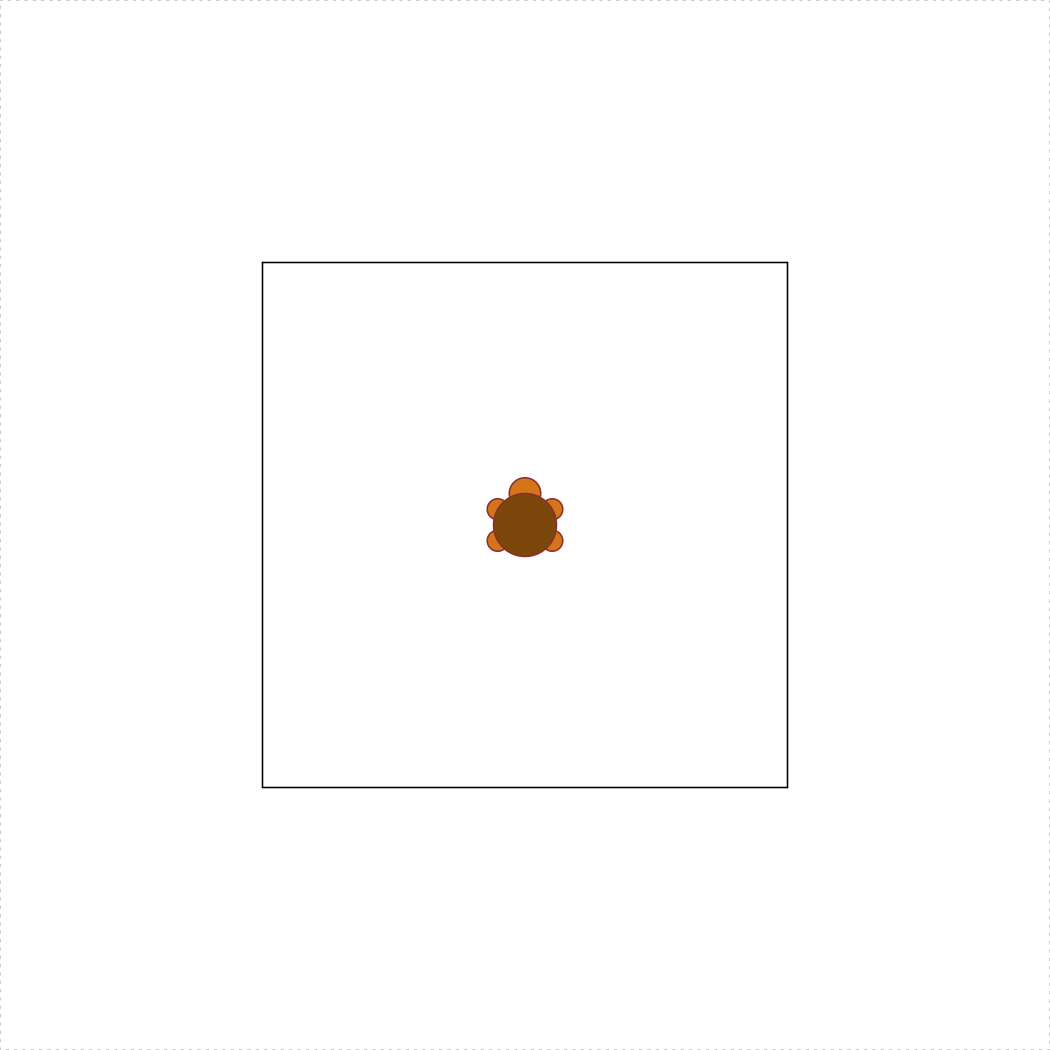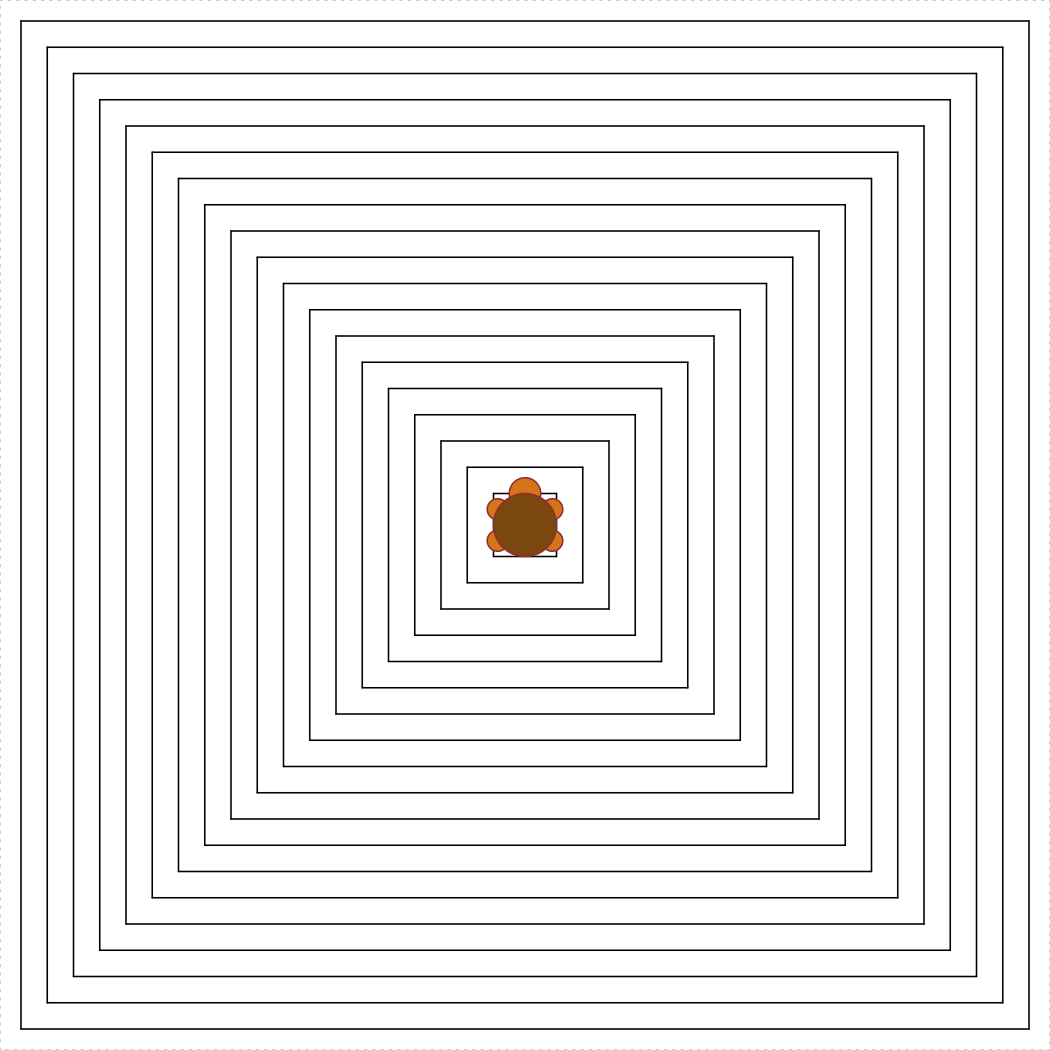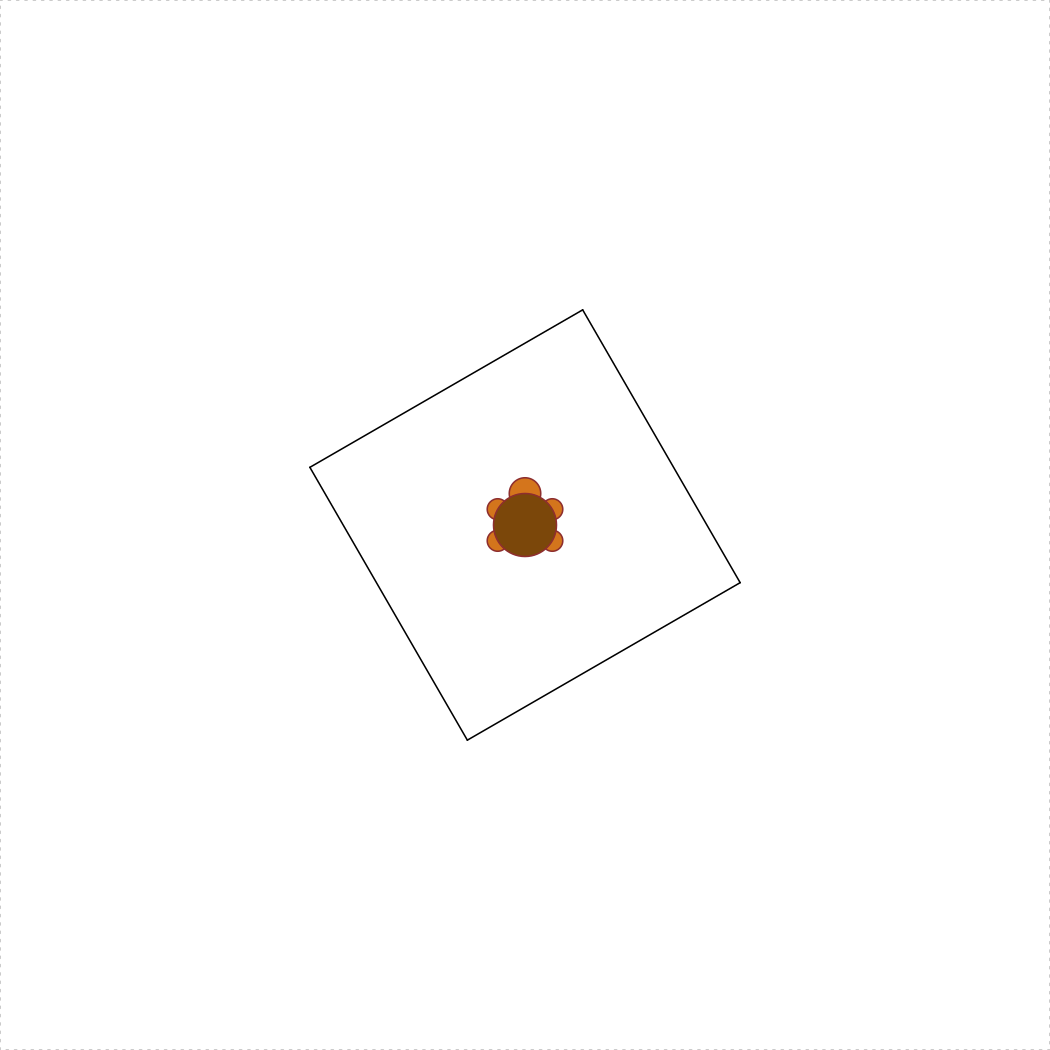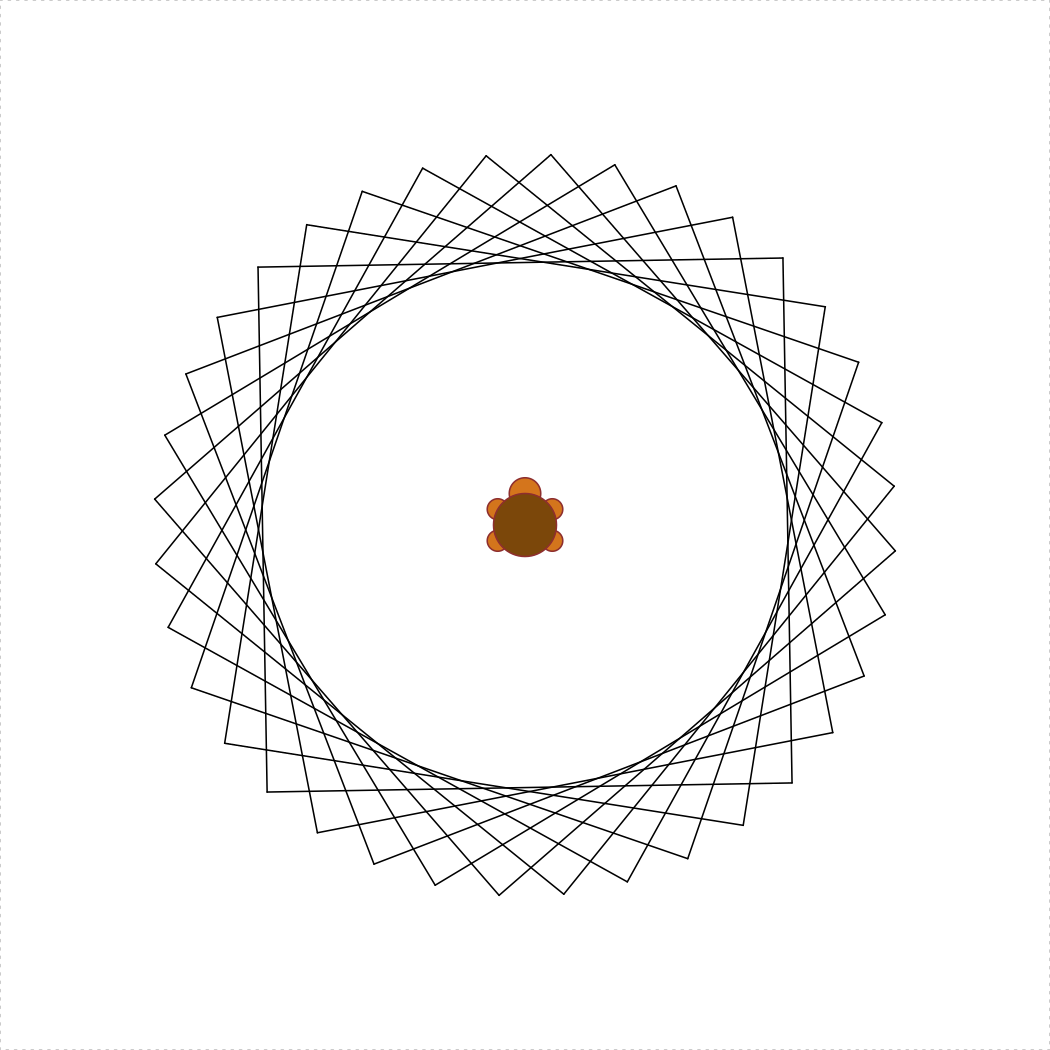Due: 05 October by 11:00 pm

Purpose, Skills, & Knowledge: The purposes of this assignment are:

• To practice using for and while loops in R.
• To practice computational problem solving with loops.

Assessment: Each question indicates the % of the assignment grade, summing to 100%. The credit for each question will be assigned as follows:

• 0% for not attempting a response.
• 50% for attempting the question but with major errors.
• 75% for attempting the question but with minor errors.
• 100% for correctly answering the question.

Rules:

• Problems marked SOLO may not be worked on with other classmates, though you may consult instructors for help.
• For problems marked COLLABORATIVE, you may work in groups of up to 3 students who are in this course this semester. You may not split up the work – everyone must work on every problem. And you may not simply copy any code but rather truly work together.
• Even though you work collaboratively, you still must submit your own solutions.

### 1) Staying organized [SOLO, 5%]

Download and use this template for your assignment. Inside the “hw5” folder, open and edit the R script called “hw5.R” and fill out your name, GW Net ID, and the names of anyone you worked with on this assignment.

### Writing test functions

For each of the following functions, write a test function first, and then write the function. Your test functions will count for half of the available credit for each problem. Think carefully about the test cases to include in your test functions.

### 2) loopFactorial(n) [SOLO, 10%]

Use a for loop to write the function loopFactorial(n) that should return n!, i.e. “n factorial”, which is defined for all non-negative integers. For example, 3! = 3*2*1 = 6, 4! = 4*3*2*1 = 24, and 5! = 5*4*3*2*1 = 120. Note that 0 is a special case, and 0! = 1. Assume n >= 0.

### 3) numDigits(n) [SOLO, 15%]

Write the function numDigits(n) that takes a possibly-negative integer and returns the number of digits in it. So, numDigits(12345) returns 5, numDigits(0) returns 1, and numDigits(-111) returns 3. One way you could solve this is to convert n to a string and use str_length(), but you cannot do that since you may not use strings here.

## Happy Numbers [COLLABORATIVE]

Background: Read the first paragraph from the Wikipedia page on happy numbers. After some thought, we see that no matter what number we start with, when we keep replacing the number by the sum of the squares of its digits, we’ll always either arrive at 4 (unhappy) or at 1 (happy). With that in mind, we want to write the function nthHappyNumber(n). However, to write that function, we’ll first need to write isHappyNumber(n), which determines whether a number is “happy” or not. And to right that function, we’ll first need to write sumOfSquaresOfDigits(n). And that’s top-down design! Here we go…

## 4) sumOfSquaresOfDigits(n) [10%]

Write the function sumOfSquaresOfDigits(n) which takes a non-negative integer, n, and returns the sum of the squares of its digits (assume that n will always be a positive integer, so no need to check for bad inputs).

## 5) isHappyNumber(n) [10%]

Write the function isHappyNumber(n) which takes a possibly-negative integer and returns TRUE if it is happy and FALSE otherwise. Note that all numbers less than 1 are not happy.

## 6) nthHappyNumber(n) [15%]

Write the function nthHappyNumber(n) which takes a non-negative integer, n, and returns the nth happy number, where nthHappyNumber(1) returns the first happy number (1).

## Turtle loops! [COLLABORATIVE]

(Note: the autograder won’t test these functions)

## 7) turtleSquare(s)redux [10%]

Re-write the turtleSquare(s) function from HW2, but this time use a for loop to draw the sides of the square. The following code should produce a square with a side length of 50:

library(TurtleGraphics)
turtle_init()
turtle_do({
turtleSquare(50)
})## 8) concentricTurtleSquares(spacing = 5) [15%]

Write the function concentricTurtleSquares(spacing) that uses the TurtleGraphics library to draw concentric squares from the center of the terrarium and outward. The spacing argument determines the spacing between each square, and the default value should be spacing = 5. Also, spacing >= 1, and your function must not allow the turtle to escape the terrarium. Hint: you may want to use turtleSquare(s) as a helper function. The following code should produce concentric squares with a spacing of 5:

library(TurtleGraphics)
turtle_init()
turtle_do({
concentricTurtleSquares(5)
})### 9) Submit your files [SOLO, 5%]

Create a zip file of all the files in your R project folder for this assignment and submit the zip file on Blackboard (note: to receive full credit, your submission must follow the above format of using a correctly-named R Project and .R script).

(Note: the autograder won’t test these functions)

### Bonus 1) turtleSquareRotated(s, degrees) [SOLO, 2.5%]

Write the function turtleSquareRotated(s, degrees) that uses the TurtleGraphics library to draw a square with side length s < 100 and rotated by degrees <= 180 counterclockwise from the horizontal plane. The rotated square should be centered in the turtle’s terrarium. Hint: you’re going to need to use the cosine (cos()) and sine (sin()) functions; in R, these functions take angles in radians (not degrees), so remember to convert your angles (180 degrees = $$\pi$$). The following code should produce a square with a side length of 30 and rotated by 30 degrees:

library(TurtleGraphics)
turtle_init()
turtle_do({
turtleSquareRotated(30, 30)
})### Bonus 2) turtleSquareStar(s, degreeSpacing = 20) [SOLO, 2.5%]

Write the function turtleSquareStar(s, degreeSpacing) that uses the turtleSquareRotated(s, degrees) as a helper function to draw a sequence of overlapping rotated squares with side length s < 70 to form a star. The degreeSpacing argument determines the spacing in degrees between each rotated square, and the default value should be degreeSpacing = 20. Also, 1 <= degreeSpacing <= 60, and your function must not allow the turtle to escape the terrarium. The following code should produce the star of rotated squares with a side length of 50 and 20 degree spacings between each square:

library(TurtleGraphics)
turtle_init()
turtle_do({
turtleSquareStar(50, 20)
})EMSE 4574: Programming for Analytics (Fall 2020) |
Tuesdays | 12:45 - 3:15 PM | Dr. John Paul Helveston | jph@gwu.edu
Content 2020 John Paul Helveston. See the licensing page for details.